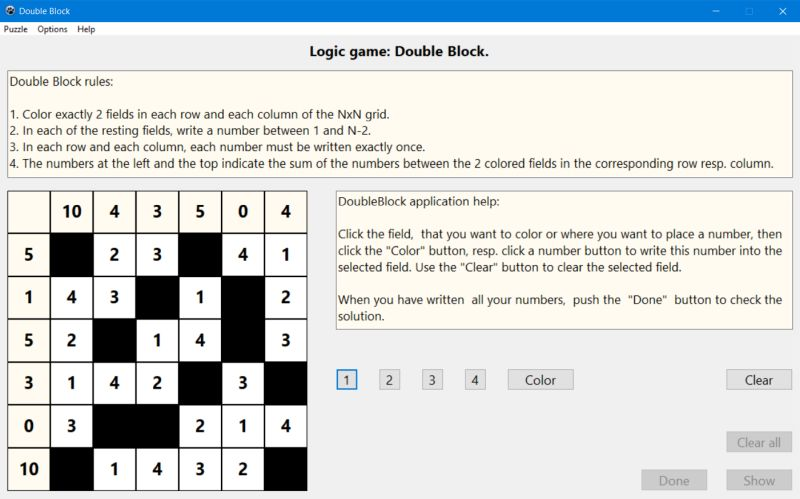# Computing: Free Pascal Programming

## Logic number puzzle: Double Block.

Description: Simple puzzle game with numbers to be placed onto a grid. The rules of the game are as follows:
1. Color exactly 2 fields in each row and each column of the N×N grid.
2. In each of the resting fields, write a number between 1 and N-2.
3. In each row and each column, each number must be written exactly once.
4. The numbers at the left and the top indicate the sum of the numbers between the 2 colored fields in the corr. row/column.
The game is a typical logic game: In order to find the correct solution of the puzzle, you have to explore the sum values, and by logical thinking (concluding from what you know, that a given field must be a colored or a given number field, or on the contrary, can't be so), determine, which are the colored fields and which numbers have to be written to the other fields.
The game is played, using the mouse: Click the field, that you want to color or where you want to write a number, then click the Color button to color it or one of the 4 number buttons to write the corresponding number. Use the Clear button to reset the actually selected field.

Improvement: The code, that generates the random grid is far from optimal and thus, the grid creation (especially with a 6×6 grid) takes more time, than it should.

Free Pascal features: Changing the color of shapes and the caption of static-texts during runtime. Using buttons to insert characters into a given control's caption. Two-dimensional arrays (classic Pascal).

Screenshot: